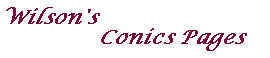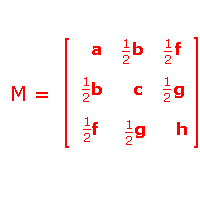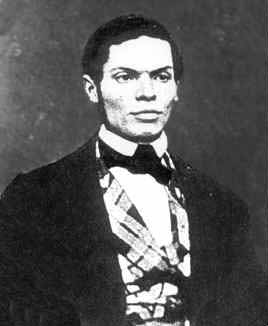Poles, polars and duality -the algebraic version

In this section, we give an algebraic treatment of these topics.
The proofs may be obtained by clicking on the link below the statement of each theorem.A plane conic has an equation of the form ax2+bxy +cy2+fx+gy+h=0.
In terms of homogeneous coordinates, this becomes ax2+bxy +cy2+fxz+gyz+hz2=0
which can be written as xTMx=0 where x=(x,y,z), and M is a symmetric 3x3 matrix.

For a non-degenerate conic, M must be non-singular and have eigenvalues of different sign.

Note that, if a conic contains three (distinct) collinear points, then it must be degenerate.

Definition
If C: xTMx=0 is a non-degenerate conic and U=[u] is any point,
then the algebraic polar of U with respect to C is the line uTMx=0.

Note that, as M is non-singular, we cannot have uTM=0, so that the line always exists.

A line L has an equation aTx=0. Now, uTMx=0 and aTx=0 give the same line if and only if [u]=[M-1a].
Thus L is the polar of a unique point U=[u].

Definition
If C: xTMx=0 is a non-degenerate conic and L is any line,
then the algebraic pole of L with respect to C is the point U=[u] such that L has equation uTMx=0.

Remark
If L has equation aTx=0, then, as we have seen, the pole of L is U=[M-1a].

Theorem 1
If C: xTMx=0 is a non-degenerate conic and U is any point on C,
then the algebraic polar of U with respect to C is the tangent to C at U .

We now show that the algebraic polar coincides with the geometrical polar.

Theorem 2
Suppose that C: xTMx=0 is a non-degenerate conic and that VW is a chord of C passing through a fixed point U.
Then the tangents at V and W meet on the algebraic polar of U with respect to C.

It follows readily that the algebraic and geometric poles of a line coincide.

We now have the idea of duality defined in algebraic terms.
The fundamental result is that duality preserves incidence.

Theorem 3 La Hire's Theorem
Suppose that C: xTMx=0 is a non-degenerate conic and that U and V are any points.
Then U lies on the polar of V if and only if V lies on the polar of U.

From certain points in the plane, it is possible to draw tangents to the conic C.
Provided the point does not lie on the conic, there will be exactly two tangents, as the following theorem shows.

Theorem 4
Suppose that C: xTMx=0 is a non-degenerate conic and that U is any point.
Then the pair of tangents to C from U has equation uTMu xTMx =uTMx uTMx .

Together, Theorems 1, 2 and 4 establishJoachimsthal's Formulae
Suppose that C: xTMx=0 is a non-degenerate conic. Then

• The polar of U=[u] has equation uTMx=0.
• If U=[u] is on C, then the tangent to C at U has equation uTMx=0.
• The pair of tangents to C from U=[u] has equation uTMu xTMx =uTMx uTMx .